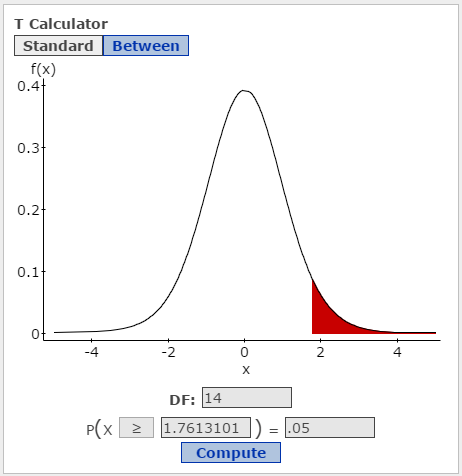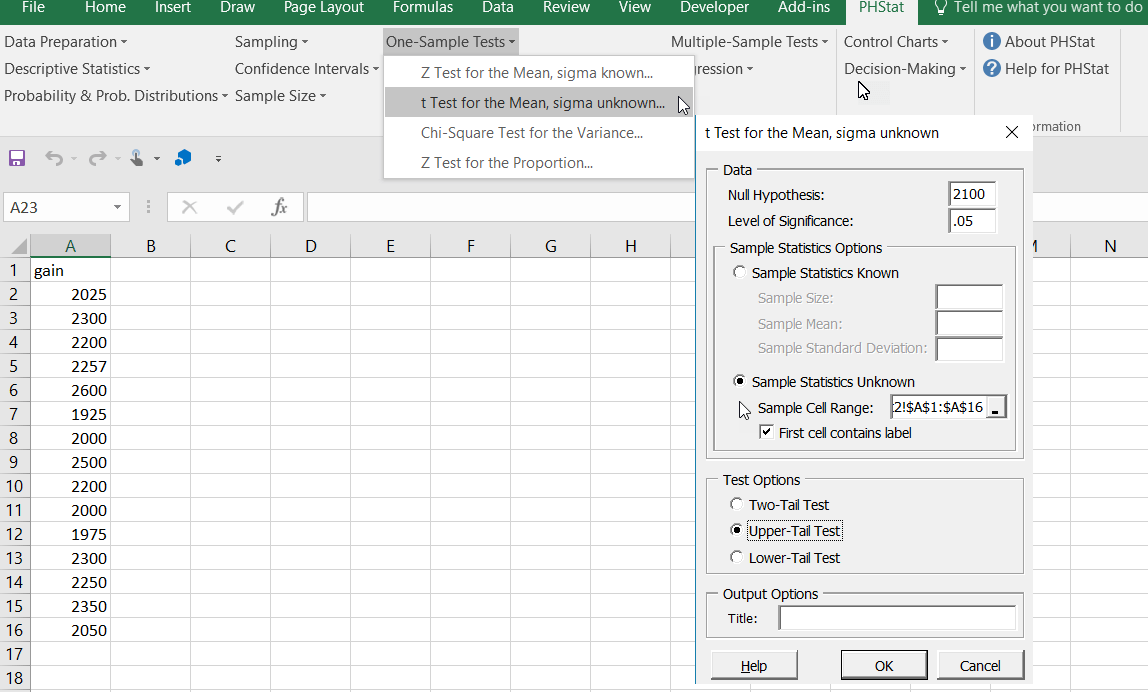# Recognizing problem types: Hypothesis tests

Read the problem looking for keywords and values:

• What type of variable is the focus of the problem? Is it quantitative, e.g. a mean, or categorical, e.g. a proportion or percent?
• How many variables are of concern?
• Is(are) the population(s) standard deviation, sigma, given?
• Are sample variances equal or are you instructed to assume they are equal?
• What is(are) the sample size(s)?
• Is a claim or proposition mentioned?
• Are you given summary statistics or raw data?

Example 1:

At many golf clubs, a teaching professional provides a free 10-minute lesson to new customers. A golf magazine reports that golf facilities that provide these free lessons gain, on average, \$2100 in green fees, lessons, or equipment expenditures. A teaching professional believes that the average gain exceeds 2,100, and collects data (shown below in the solution examples) on the gain from 15 clubs in his area. At the 0.05 level of significance, does the data support the claim?

What should you glean from reading the problem statement?

• This is a single-sample test about a mean.
• The teaching professional believes that average gain exceeds \$2100. Thus the claim is that the mean gain exceeds \$2100. The phrase “exceeds \$2100” indicates the claim contains the “>” math operator which is a form of inequality. Thus, the claim must be the alternative hypothesis, Ha: μ > \$2100, because the null must be an equality.
• The null hypothesis is always the complement of Ha and is Ho: μ <= \$2100.
• Because Ha contains the > operator, this is a right-tailed test. Remember the mnemonic that the math operator in the alternative points toward the tail of the test.
• We are given raw data, N = 15, and we do not know the population standard deviation, sigma. Because we do not know sigma and N is less than 30, we must use a t-test for a small sample.
• The degrees of freedom for the t-test are N-1 or 14.

Using the StatCrunch t-distribution calculator [Excel:  =-T.INV(.05,14)], the critical value of t for a right-tail test with an alpha of 0.05 is 1.76.• If the t-test statistic for the data is greater than 1.76 (or p<0.05), we reject the null and accept the alternative.
• Using technology, see below, the test statistic is 1.85 which is greater than 1.76 and falls in the rejection region. Accordingly, the p-value is 0.0425 which is less than alpha = 0.05. Both methods indicate the null hypothesis should be rejected in favor of the alternative.
• Because the claim is the alternative, which has been supported, the conclusion is “There is sufficient evidence to support the claim that the average gain from giving free lessons to new customers is greater than \$2100.”

Example solutions:

StatCrunch: The data is in column “gain.” 1. Select Stat>T Stats>One Sample>With Data. 2. In the One Sample T dialog box (2), click on “gain” in the Select Column(s) section, select the Perform Hypothesis test for µ radio button. Enter the null hypothesis, Ho: μ= 2100. Select > in the Ha: μ > 2100 and click Compute. 3. The results of the hypothesis test are in the box (with Options button) that opens. The t-test statistic is 1.85 and the p-value is 0.0425.Excel PHStat:

The data is in column A. Select PHSTAT>One-Sample Tests>t-test for Mean, sigma unknown. In the dialog box that opens, enter the Null Hypothesis of 2100, the Level of Significance of 0.05, click on Sample Statistics Unknown, enter the range of the data values, check that the First cell contains a label, select an Upper-tail Test and click OK.A new tab will be inserted with the name Hypothesis. The t-test statistic is 1.85 and the p-value is 0.0425.This site uses Akismet to reduce spam. Learn how your comment data is processed.